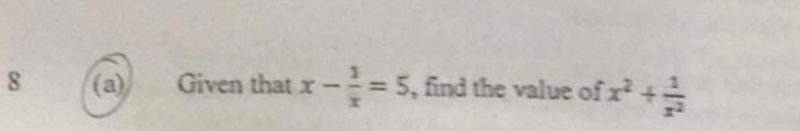QuestionI am not a teacher and my maths may be a little rusty. However, cannot resist to give this a try. Please have a look and let me know if there is any mistake with my working. Its been a long time after all…..

x – (1/x) = 5

Taking the square of both sides

[x – (1/x)] 2= 25

x2 – 2(x) (1/x) + (1/x)2 = 25

x2 – 2 + 1/x2 = 25

x2 + 1/x2 = 25 + 2

x2 + 1/x2 = 27

0 Replies 0 Likes